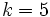# Abelian normal subgroup of group of prime power order

This article describes a property that arises as the conjunction of a subgroup property: abelian normal subgroup with a group property imposed on the ambient group: group of prime power order
View a complete list of such conjunctions | View a complete list of conjunctions where the group property is imposed on the subgroup

## Definition

Suppose$G$ is a group and$H$ is a subgroup of$G$. We say that$H$ is an abelian normal subgroup of group of prime power order if$H$ is an abelian normal subgroup of$G$ and$G$ is a group of prime power order (i.e., a finite p-group for some prime number$p$).

## Facts

### Existence, congruence conditions, and replacement

In the points below,$p$ is a prime number.

• It turns out that for any$k$, there exists some large enough$n_0$ such that for$n \ge n_0$, any group of order$p^n$ contains an abelian normal subgroup of order$p^k$. Specifically, we can choose$n_0 = k(k-1)/2 + 1$, though smaller values of$n_0$ may also work. Further information: Existence of abelian normal subgroups of small prime power order
• In many cases, if an abelian subgroup of a given order exists, the number of abelian normal subgroups of that order in a finite$p$-group is congruent to$1$ modulo$p$. For$p$ odd, this holds for order$p^k, 0 \le k \le 5$, in any$p$-group that contains at least one abelian normal subgroup of order$p^k$. This is the Jonah-Konvisser congruence condition on number of abelian subgroups of small prime power order. For$p = 2$, the result holds for$0 \le k \le 4$, though it is open for$k = 5$. In such situations, we say that the collection of abelian groups of that particular order is a collection of groups satisfying a universal congruence condition.
• In an even wider range of cases, if an abelian subgroup of a given order exists, so does an abelian normal subgroup. In this case, we say that the abelian groups of that particular order satisfy a weak normal replacement condition. A stronger version is that an abelian normal subgroup exists that is contained in the normal closure of the original abelian subgroup. In this case, we say that the abelian groups of that particular order satisfy a strong normal replacement condition.
• There is a wide range of results called replacement theorems, that aim to replace one abelian subgroup by another, the latter often being normal.